Investment-Cost Optimization of Plastic Recycling System under Reliability Constraints

2Jilali Liabes University, Electrical Department, Sidi Bel Abbes, Algeria

E-mail: azeblah@yahoo.fr

Abstract

This paper describes and uses an ant colony meta-heuristic optimization method to solve the redundancy optimization problem in plastic recycling industry. This problem is known as total investment-cost minimization of series-parallel plastic recycling system. Redundant components are included to achieve a desired level of availability. System availability is represented by a multi-state availability function. The plastic machines are characterized by their capacity, availability and cost. These machines are chosen among a list of products available on the market. The proposed meta-heuristic seeks to find the best minimal cost plastic recycling system configuration with desired availability. To estimate the series-parallel plastic machines availability, a fast method based on universal moment generating function (UMGF) is suggested. The ant colony approach is used as an optimization technique. An example of plastic recycling system is presented.

Keywords

Ant Colony; Redundancy Optimization; Multi-state Systems; Universal Generating Function (UMGF); Plastic Recycling.

Introduction

One of the most important problems in many plastic industrial applications is the redundancy optimization problem. This latter is well known combinatorial optimization problem where the design goal is achieved by discrete choices made from components available on the market. The natural objective function is to find the minimal cost configuration of a series-parallel plastic recycling system under availability constraints. The system is considered to have a range of performance levels from perfect working to total failure. In this case the system is called a multi-state system (MSS). Let consider a multi-state recycling system containing n subsystems Ci (i = 1, 2, …, n) in series arrangement. For each subsystem Ci there are various versions, which are proposed by the suppliers on the market. Plastic components are characterized by their cost, capacity and availability according to their version. For example, these components can represent machines in a recycling system to accomplish a task on product in our case they represent the chain of plastic transformation (conveyors, grinders, electrostatic separators and electric furnaces). Each subsystem Ci contains a number of machines connected in parallel. Different versions of components may be chosen for any given subsystem. Each subsystem can contain machines of different versions as sketched in figure 1.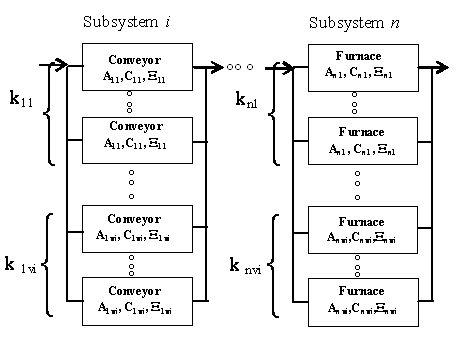Figure 1. Series-parallel plastic recycling system structure

Literature Review

The vast majority of classical reliability or availability analysis and optimization assume that components and system are in either of two states (i.e., complete working state and total failure state). However, in many real life situations we are actually able to distinguish among various levels of performance (capacity) for both system and components. For such situation, the existing dichotomous model is a gross oversimplification and so models assuming multi-state (degradable) systems and components are preferable since they are closer to reliability. Recently much works treat the more sophisticated and more realistic models in which systems and components may assume many states ranging from perfect functioning to complete failure. In this case, it is important to develop MSS reliability theory. In this paper, an MSS reliability theory will be used, where the binary state system theory is extending to the multi-state case. As is addresses in recent review of the literature for example in [1, 2]. Generally, the methods of MSS reliability assessment are based on four different approaches:

(i)              The structure function approach.

(ii)            The stochastic process (mainly Markov) approach.

(iii)           The Monte-Carlo simulation technique.

(iv)          The universal moment generating function (UMGF) approach.

In reference , a comparison between these four approaches highlights that the UMGF approach is fast enough to be used in the optimization problems where the search space is sizeable.

The problem of total investment-cost minimization, subject to reliability or availability constraints, is well known as the redundancy optimization problem (ROP). The ROP is studied in many different forms as summarized in , and more recently in . The ROP for the multi-state reliability was introduced in . In  and , genetic algorithms were used to find the optimal or nearly optimal transformation system structure.

This work uses an ant colony optimization approach to solve the ROP for multi-state plastic recycling system. The idea of employing a colony of cooperating agents to solve combinatorial optimization problems was recently proposed in . The ant colony approach has been successfully applied to the classical traveling salesman problem in , and to the quadratic assignment problem in . Ant colony shows very good results in each applied area. It has been recently adapted for the reliability design of binary state systems in . The ant colony has also been adapted with success to other combinatorial optimization problems such as the vehicle routing problem in . The ant colony method has not yet been used for the redundancy optimization of multi-state systems.

Approach and Outlines

The problem formulated in this paper lead to a complicated combinatorial optimization problem. The total number of different solution to be examined is very large, even for rather small problems. An exhaustive examination of all possible solutions is not feasible given reasonable time limitations. Because of this, the ant colony optimization (or simply ACO) approach is adapted to find optimal or nearly optimal solutions to be obtained in a short time. The newer developed meta-heuristic method has the advantage to solve the ROP for MSS without the limitation on the diversity of versions of components in parallel.

During the optimization process, artificial ants will have to evaluate the availability of a given selected structure of the series-parallel plastic recycling system. To do this, a fast procedure of availability estimation is developed. This procedure is based on a modern mathematical technique: the z-transform or UMGF which was introduced in . It was proven to be very effective for high dimension combinatorial problems: see e.g., in . The universal moment generating function is an extension of the ordinary moment generating function (UGF) in . The method developed in this paper allows the availability function of reparable series-parallel MSS to be obtained using a straightforward numerical procedure.

The rest of this paper is outlined as follows. We start in section 2 with the formulation of the optimization problem of plastic recycling. In section 3, we develop the availability estimation of a series-parallel multi-state recycling system method. In section 4, we describe the ant colony optimization approach to solve the redundancy optimization problem of plastic recycling industry. In section 5, illustrative examples and numerical results are presented in which the optimal choice of machines in a system is found. Conclusions are drawn in section 6.

Formulation of the optimization problem of plastic recycling

Let consider a series-parallel recycling system containing n subsystems Ci (i = 1, 2, …, n) in series arrangement as represented in figure 1. Every subsystem Ci contains a number of different machines connected in parallel. For each subsystem i, there are a number of machine versions available in the market. For any given system machine, different versions and number of machines may be chosen. For each subsystem i, machines are characterized according to their version v by their cost (Civ), availability (Aiv) and capacity (Σiv). The structure of subsystem i can be defined by the numbers of parallel machines (of each version) kiv for 1≤ v ≤Vi, where Vi is a number of versions available for machines of type i. Figure 2 illustrates these notations for a given subsystem i. The entire system structure is defined by the vectors ki = {kiv} (1 ≤ i ≤ n, 1 ≤ v ≤ Vi). For a given set of vectors k1, k2, …, kn the total cost of the system (C) can be calculated as: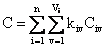(1)

Availability of reparable multi-state recycling systems

The series-parallel recycling system is composed of a number of failure prone machines, such that the failure of some machines leads only to a degradation of the system performance. This system is considered to have a range of performance levels from perfect working to complete failure. In fact, the system failure can lead to decreased capability to accomplish a given task, but not to complete failure. An important MSS measure is related to the ability of the system to satisfy a given demand.

For instance in electric power systems, reliability is considered as a measure of the ability of the system to meet the load demand (D), i.e., to provide an adequate supply of electrical energy (Σ). This definition of the reliability index is widely used in power systems: see e.g., in [14-16] and in [6-7]. The Loss of Load Probability index (LOLP) is usually used to estimate the reliability index in . This index is the overall probability that the load demand will not be met. Thus, we can write R = P ³ D) or R = 1-LOLP with LOLP = P < D). This reliability index depends on consumer demand D.

For reparable MSS, a multi-state steady-state availability E is used as P ³ D) after enough time has passed for this probability to become constant in . In the steady-state the distribution of states probabilities is given by equation (2), while the multi-state stationary availability is formulated by equation (3):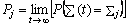(2)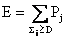(3)

If the operation period T is divided into M intervals (with duration's T1, T2, …, TM) and each interval has a required demand level (D1, D2, …, DM , respectively), then the generalized MSS availability index A is: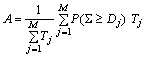(4)

We denote by D and T the vectors {Dj} and {Tj}(1 ≤ j ≤ M), respectively. As the availability A is a function of k1, k2,…, kn, D and T, it will be written A(k1, k2, …, kn, D, T). In the case of a power system, the vectors D and T define the cumulative load curve (consumer demand). In reality the load curves varies randomly; an approximation is used from random curve to discrete curve see in . In general, this curve is known for every power system.

Optimal design problem formulation

The multi-state plastic recycling system redundancy optimization problem can be formulated as follows: find the minimal cost system configuration k1, k2, …, kn, such that the corresponding availability exceeds or equal the specified availability A0. That is,

Minimize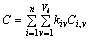(5)

subject to

 A(k1, k2, …, kn, D, T) ³ A0 (6)

The input of this problem is the specified availability and the outputs are the minimal investment-cost and the corresponding recycling configuration. To solve this combinatorial optimization problem, it is important to have an effective and fast procedure to evaluate the availability index for a series-parallel plastic recycling system. Thus, a method is developed in the next section to estimate the value of A(k1, k2, …, kn, D, T).

Multi-state system availability estimation method

The procedure used in this paper is based on the universal z-transform, which is a modern mathematical technique introduced in . This method, convenient for numerical implementation, is proved to be very effective for high dimension combinatorial problems. In the literature, the universal z-transform is also called universal moment generating function (UMGF) or simply u-function or u-transform. In this paper, we mainly use the acronym UMGF. The UMGF extends the widely known ordinary moment generating function in .

Definition

The UMGF of a discrete random variable Σ is defined as a polynomial: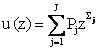(7)

where the variable Σ has J possible values and Pj is the probability that Σ is equal to Σj.

The probabilistic characteristics of the random variable Σ can be found using the function u(z). In particular, if the discrete random variable Σ is the MSS stationary output performance, the availability E is given by the probability P ³ D) which can be defined as follows: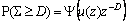(8)

where ψ is a distributive operator defined by expressions (9) and (10):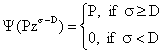(9)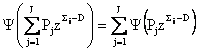(10)

It can be easily shown that equations (7)-(10) meet condition

 P(Σ ³ D) =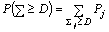By using the operator Ψ, the coefficients of polynomial u(z) are summed for every term with Σj ³ D, and the probability that Σ is not less than some arbitrary value D is systematically obtained. Consider single components with total failures and each component i has nominal performance Σi and availability Ai. Then, P(Σ = Σi) = Ai and P(Σ = 0) = 1 - Ai. The UMGF of such an component has only two terms can be defined as: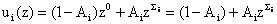(11)

To evaluate the MSS availability of a series-parallel system, two basic composition operators are introduced. These operators determine the polynomial u(z) for a group of components.

Parallel components

Let consider a subsystem m containing Jm components connected in parallel. As the performance measure is related to the system productivity, the total performance of the parallel subsystem is the sum of performances of all its components. In power systems engineering, the term capacity is usually used to indicate the quantitative performance measure of an component in . It may have different physical nature. Examples of components capacities are: generating capacity for a generator, pipeline capacity for a water circulator, carrying capacity for an electric transmission line, etc. The capacity of an component can be measured as a percentage of nominal total system capacity. In a electrical network, components are generators, transformers and electrical lines. Therefore, the total performance of the parallel component is the sum of performances in .

The u-function of MSS subsystem m containing Jm parallel components can be calculated by using the Γ operator: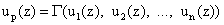where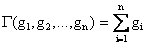Therefore for a pair of components connected in parallel: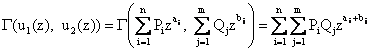Parameters ai and bj are physically interpreted as the respective performances of the two components. n and m are numbers of possible performance levels for these components. Pi and Qj are steady-state probabilities of possible performance levels for components.

One can see that the Γ operator is simply a product of the individual u-functions. Thus, the subsystem UMGF is: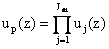Given the individual UMGF of components defined in equation (11), we have: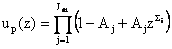Series components

When the components are connected in series, the component with the least performance becomes the bottleneck of the system. This component therefore defines the total system productivity. To calculate the u-function for system containing n subsystems connected in series, the operator η should be used: us(z) = η(u1(z), u2(z), …, um(z)) where η(g1, g2, …, gm) = min{g1, g2, …, gm}, so that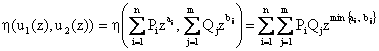Applying composition operators Γ and η consecutively, one can obtain the UMGF of the entire series-parallel system. To do this we must first determine the individual UMGF of each component.

Components with total failures

Let consider the usual case where only total failures are considered (K = 2) and each component of type i and version vi has nominal performance Σiv and availability Aiv. In this case, we have: P(Σ = Σiv) = Aiv and P(Σ = 0) = 1 - Aiv. The UMGF of such an component has only two terms and can be defined as in equation (11) by u*i(z) = (1-Aiv)z0 + Aivziv = 1 – Aiv + Aivziv.

Using the Γ operator, we can obtain the UMGF of the i-th system component containing ki parallel components as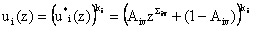The UMGF of the entire system containing n components connected in series is: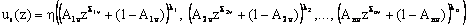(12)

To evaluate the probability P(Σ ³ D) for the entire system, the operator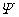is applied to equation (12):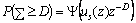(13)

The above procedure was implemented and tested on a PC computer and shown to be effective and fast. The UMGF method, convenient for numerical implementation, is efficient for the high dimension combinatorial problem formulated in this work. In our optimization technique to solve this problem, artificial ants will evaluate the availability of given selected structures of the series-parallel recycling system. To do this, the fast implemented procedure of availability estimation will be used by the optimization program.

The next section presents the ant colony meta-heuristic optimization method to solve the redundancy optimization problem for multi-state plastic recycling systems.

The ant colony optimization approach

The problem formulated in this paper is a complicated combinatorial optimization problem. The total number of different solutions to be examined is very large, even for rather small problems. An exhaustive examination of the enormous number of possible solutions is not feasible given reasonable time limitations. Thus, because of the search space size of the ROP for MSS, a new meta-heuristic is developed in this section. This meta-heuristic consists in an adaptation of the ant colony optimization method.

The ACO principle

Recently, in  introduced a new approach to optimization problems derived from the study of any colonies, called “Ant System”. Their system inspired by the work of real ant colonies that exhibit the highly structured behavior. Ants lay down in some quantity an aromatic substance, known as pheromone, in their way to food. An ant chooses a specific path in correlation with the intensity of the pheromone. The pheromone trail evaporates over time if no more pheromone in laid down by others ants, therefore the best paths has more intensive pheromone and higher probability to be chosen. This simple behavior explains why ants are able to adjust to changes in the environment, such as new obstacles interrupting the currently shortest path.

Artificial ants used in ant system are agents with very simple basic capabilities mimic the behavior of real ants to some extent. This approach provides algorithms called ant algorithms. The Ant System approach associates pheromone trails to features of the solutions of a combinatorial problem, which can be seen as a kind of adaptive memory of the previous solutions. Solutions are iteratively constructed in a randomized heuristic fashion biased by the pheromone trails, left by the previous ants. The pheromone trails, tij , are updated after the construction of a solution, enforcing that the best features will have a more intensive pheromone. An Ant algorithm presents the following characteristics. It is a natural algorithm since it is based on the behavior of ants in establishing paths from their colony to feeding sources and back. It is parallel and distributed since it concerns a population of agents moving simultaneously, independently and without supervisor. It is cooperative since each agent chooses a path on the basis of the information, pheromone trails, laid by the other agents with have previously selected the same path. It is versatile that can be applied to similar versions the same problem. It is robust that it can be applied with minimal changes to other combinatorial optimization problems. The solution of the travelling salesman problem (TSP) was one of the first applications of ACO.

Various extensions to the basic TSP algorithm were proposed, notably by Dorigo and Gambardella in . The improvements include three main aspects: the state transition rule provides a direct way to balance between exploration of new edges and exploitation of a priori and accumulated knowledge about the problem, the global updating rule is applied only to edges which belong to the best ant tour and while ants construct solution, a local pheromone updating rule is applied.  These extensions have been included in the algorithm proposed in this paper.

ACO-based solution approach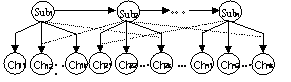with n subsystems into a graph

In figure 2, a series-parallel recycling system is illustrated. At each step of the construction process, an ant uses problem-specific heuristic information, denoted by hij to choose the optimal number of components in each subsystem. Imaginary heuristic information is associated to each blank node.  These new factors allow us to limit the search surfaces (i.e. tuning factors). An ant positioned on subsystem i chooses a component j by applying the rule given by: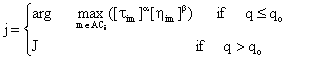(14)

and J is chosen according to the probability:(15)

a :       The relative importance of the trail.

b :        The relative importance of the heuristic information hij.

ACi:     The set of available components choices for subsystem i.

q:         Random number uniformly generated between 0 and 1.

The heuristic information used is : hij = 1/(1+cij) where cij represents the associated cost of component j for subsystem i. A “tuning” factor ti= hij = 1/(1+ci(Mi+1)) is associated to blank component (Mi+1) of subsystem i. The parameter qo determines the relative importance of exploitation versus exploration: every time an ant in subsystem i have to choose a component j, it samples a random number 0≤q≤1. If qqo then the best edge, according to (14), is chosen (exploitation), otherwise an edge is chosen according to (15) (biased exploration).

The pheromone update consists of two phases: local and global updating. While building a solution of the problem, ants choose components and change the pheromone level on subsystem-component edges. This local trail update is introduced to avoid premature convergence and effects a temporary reduction in the quantity of pheromone for a given subsystem-component edge so as to discourage the next ant from choosing the same component during the same cycle. The local updating is given by: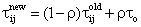(16)

where r is a coefficient such that (1-r) represents the evaporation of trail and to is an initial value of trail intensity. It is initialized to the value (n.TCnn)-1 with n is the size of the problem (i.e. number of subsystem and total number of available components) and TCnn is the result of a solution obtained through some simple heuristic.

After all ants have constructed a complete system, the pheromone trail is then updated at the end of a cycle (i.e. global updating), but only for the best solution found. This choice, together with the use of the pseudo-random-proportional rule given by (14) and (15), is intended to make the search more directed: ants search in a neighborhood of the best solution found up to the current iteration of the algorithm. The pheromone level is updated by applying the following global updating rule: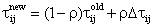(17)(18)

The Ant algorithm

An ant-cycle algorithm is stated as follows. At time zero an initialization phase takes place during wish NbAnt ants select components in each subsystem according to the Pseudo-random-proportional transition rule given by (14) and (15). When an ant selects a component, a local update is made to the trail for that subsystem-component edge according to equation (16). In this equation, r is a parameter that determines the rate of reduction of the pheromone level. The pheromone reduction is small but sufficient to lower the attractiveness of precedent subsystem-component edge. At the end of a cycle, for each ant k, the value of the system’s reliability Ak and the total cost TCk are computed. The best feasible solution found by ants (i.e. total cost and assignments) is saved. The pheromone trail is then updated for the best solution obtained according to (17) and (18). This process is iterated until the tour counter reaches the maximum number of cycles NCmax or all ants make the same tour (stagnation behavior).

Illustrative Example

In order to illustrate the proposed ant colony algorithm, a plastic recycling system is considered.

The station plastic feeding system (raw material) supplies the electric furnace. It consists of five basic subsystems (type of machines).

1-     Conveyor1 witch loads the plastic to the grinder where it is grinded.

2-     The grinders grind the plastic and supply it to the electro static separators.

3-     The electro separators separate the different type of plastic and then lift the different kinds of plastic up to the second conveyors.

4-     The second conveyor supplies the electric furnaces.

5-     In the furnace the transformation occurs from the solid form to fluid form until finished product.

Table 1 shows the numerical data for each recycling machines. Each recycling machine of the subsystem is considered as a unit with total failures. Table 2 contains the data of cumulative demand.

Table 1. Data examples. Subsystem Availability A Cost C Capacity S

 Subsystem Availability A Cost C Capacity S Conveyor 1 2 3 4 0.980 0.977 0.982 0.978 0.590 0.535 0.470 0.420 120 100 85 85 Grinder 1 2 3 4 5 0.995 0.996 0.997 0.997 0.998 0.205 0.189 0.091 0.056 0.042 100 92 53 28 21 Electro separator 1 2 3 4 0.971 0.973 0.971 0.976 7.525 4.720 3.590 2.420 100 60 40 20 Electric Furnace 1 2 3 4 0.984 0.983 0.987 0.981 7.525 4.720 3.590 2.420 100 60 40 20

Table 2. Parameters of the cumulative demand curve

 Demand level (%) 100 80 50 20 Duration (h) 4203 788 1228 2536 Probability 0.479 0.089 0.14 0.289

The numbers of machines Chmax in parallel are set to (4,5,4,6,4). The number of ants used to find the best solution is 30. The simulation results depend greatly on the values of the coefficients a and b. Different ti values (tuning factors associated to blank components) were tested and shown to influence greatly the algorithm. The best found values of ti are (t1= -0.13, t2= -0.04, t3= 2.3, t4= -0.35, t5= 0.35). Several simulations are made for a=5 and b=1 and the best solution is obtained in 500 cycle. Table 3 presents the obtained configuration.

Description of the system to be optimized

The plastic recycling system which supplies the consumers is designed with five basic subsystems as depicted in figure.3 and 4. The figure.1 showed the detailed process of plastic recycling system.

The process of system follows as: The conveyor 1 supplies the grinders (subsystem 1). Then grinders grind the material raw (plastic used) (subsystem 2). Then transported to electric separator by the conveyor 2 (subsystem 3). A second transportation is done by the second conveyor (subsystem 4) which supplies the electric furnace (subsystem 5). Each component of the system is considered as unit with total failures.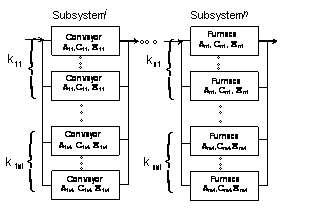Figure 3. Detailed plastic recycling system structure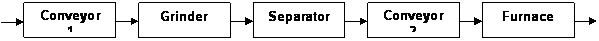Figure 4. Synoptic of plastic recycling system structure

The characteristics of the products available on the market for each type of device are presented in table 1. This table shows for each subsystem availability A, nominal capacity S and cost per unit C. With out loss of generality both the component capacity and the demand levels table 2 can be measured as a percentage of the maximum capacity.

Optimal design solution and result discussion

Our natural objective function is to define the minimal cost power system configuration which provides the requested level of availability. The whole of the results obtained by the proposed ant algorithm for different given values of A0 are illustrated in Table 3. This latter also shows the computed availability index A, the cost C of the system and their corresponding structures. Three different solutions for A0 = 0.975, A0 = 0.985, A0 = 0.995 are represented. In these experiments the values parameters of the ACO algorithm are the set of the following values: a = 5, b = 1, t0 = 0.05 and r = 0.080.The choice of these values affects strongly the solution. These values were obtained by a preliminary optimisation phase. The ACO algorithm is tested well for quite a range of these values. In the ACO algorithm 30 ants are used in each iteration. The stopping criterion is when the number of iterations attempt 500 cycles. The space search visited by the 30 ants is composed of 15000 solutions (30*500 cycles) and the huge space size of an exhaustive search (combinatorial algorithm) is not realistic. Indeed, a large comparison between the ACO and an exhaustive one, clearly the goodness of the proposed ACO meta- heuristic which respect to the calculating time.

Table 3. Optimal solutions obtained by ant colony algorithm

 A0 Optimal Structure Computed Availability A Computed Cost C 0.975 Subsystem 1: Components 1- 2 - 3 - 4 Subsystem 2: Components 1 - 2 - 3 - 4 - 5 Subsystem 3: Components 2- 3- 4 - 4 Subsystem 4: Components 1- 2 -3 - 4 -5 - 6 Subsystem 5: Components 2 - 3 - 4 - 4 0.986 18.822 0.985 Subsystem 1: Components 1 - 3 - 3 - 4 Subsystem 2: Components 1 - 2 - 3 - 4 - 5 Subsystem 3: Components 2 - 3 - 4 - 4 Subsystem 4: Components 1 - 2 - 3 - 4 - 5 - 6 Subsystem 5: Components 2 - 3 - 3 -4 0.9860 18.771 0.995 Subsystem 1: Components 2 - 3 - 4 - 4 Subsystem 2: Components 1 - 3 - 4 - 4 - 5 Subsystem 3: Components 2 - 3 - 3 - 4 Subsystem 4: Components 1 - 2 - 3 - 4 - 5 - 6 Subsystem 5: Components 1 - 2 - 3 - 4 0.998 20.199

Conclusion

A new algorithm for choosing an optimal series-parallel plastic recycling structure configuration is proposed which minimizes total investment cost subject to availability constraints. This algorithm seeks and selects machines among a list of available products according to their availability, nominal capacity (performance) and cost. Also defines the number and the kind of parallel components in each subsystem. The proposed method allows a practical way to solve wide instances of reliability optimization problem of multi-state power systems without limitation on the diversity of versions of components put in parallel. A combination is used in this algorithm is based on the universal moment generating function and an ant colony optimization algorithm.

References

1.      Ushakov, Levitin G., Lisnianski A., Multi-state system reliability: from theory to practice, Proc. of 3 Int. Conf. on mathematical methods in reliability, MMR 2002, Trondheim, Norway, 2002, p. 635-638.

2.      Levitin G., Lisnianski A, A new approach to solving problems of multi-state system reliability optimization, Quality and Reliability Engineering International, 2001, 47(2), p. 93-104.

3.      Hwang T., Tillman K.F.A., Hwang C.L., Kuo W., Optimization Techniques for System Reliability with Redundancy – A review, IEEE Transactions on Reliability, 1997, R-26(3), p. 148-155.

4.      Prasad K., An Annotated Overview of System-reliability Optimization, IEEE Transactions on Reliability, 2000, 49(2), p. 176-187.

5.      Ushakov. I, Optimal standby problems and a universal generating function, Sov. J. Computing System Science, 1987, 25(4), p. 79-82.

6.      Lisnianski. A, Levitin. G, Ben-Haim. H, Elmakis. D, Power system structure optimization subject to reliability constraints, Electric Power Systems Research, 1986, 39(2), p.145-152.

7.      Levitin. G, Lisnianski. A, Ben-Haim. H, Elmakis. D, Structure optimization of power system with different redundant components, Electric Power Systems Research, 1997, 43(1), p.19-27.

8.      Maniezzo. D, Colorni. A, The Ant System: Optimization by a colony of cooperating agents, IEEE Transactions on Systems, Man and Cybernetics- Part B, 1996, 26(1), p. 1-13.

9.      Dorigo. A, Gambardella. L, Ant Colony System: A Cooperative Learning Approach to the Traveling Salesman Problem, IEEE Transactions on Evolutionary computation, 1997, 1(1), p. 53-66.

10.  Maniezzo. D and Colorni. A, The Ant System Applied to the Quadratic Assignment Problem, IEEE Transactions on Knowledge and data Engineering, 1997, 11(5), p. 769-778.

11.  LiangY.C, and Smith. E, An Ant Colony Approach to Redundancy Allocation, IEEE Transactions on Reliability, 2001.

12.  Bullnheimer. B, Hartl. F, and Strauss. C, Applying the Ant System to the vehicle Routing problem,  2nd Metaheuristics International Conference (MIC-97), Sophia-Antipolis, France, 1997, pp. 21-24.

13.  Ushakov. I, Universal generating function, Sov. J. Computing System Science, 1996, 24(5), p. 118-129.

14.  Ross. M, Introduction to probability models, Academic Press, 1993.

15.  Murchland. J, Fundamental concepts and relations for reliability analysis of multi-state systems, Reliability and Fault Tree Analysis, ed. R. Barlow, J. Fussell, N. Singpurwalla. SIAM, Philadelphia, 1975, pp. 49-56.

16.  Levitin. G, Lisnianski. A, Ben-Haim. H, Elmakis. D, Redundancy optimization for series-parallel multi-state systems, IEEE Transactions on Reliability, 1998, 47(2), p.165-172.

17.  Billinton A., Reliability evaluation of power systems, Pitman, 1990.

18.  Wood A.J., Ringlee R.J., Frequency and duration methods for power reliability calculations, Part II, Demand capacity reserve model, IEEE Trans. on PAS, 1970, 94, p. 375-388.

19.  Dallery. S, Gershwin. Y, Manufacturing Flow Line Systems: A Review of Models and Analytical Results, Queueing Systems theory and Applications, Special Issue on Queueing Models of Manufacturing Systems, 1992, 12(1-2), p. 3-94.